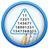# Power, Indices and SurdsQuantitative Aptitude Questions and Answers section on “Power, Indices and Surds” with solution and explanation for competitive examinations such as CAT, MBA, SSC, Bank PO, Bank Clerical and other examinations.

# Aptitude Question ID: 107146

The simplified form of is : [A] [B] [C] [D] Show Answer ∴ Expression = Hence option [B] is correct answer.

# Aptitude Question ID: 107143

If is presented in the form of perfect square it will be equal to : [A] [B] [C] [D] Show Answer Hence option [D] is correct answer.

# Aptitude Question ID: 107140

The value of is : [A] [B] [C] [D] Show Answer Expression = Hence option [C] is correct answer.

# Aptitude Question ID: 107135

Find the value of : [A]1 [B]5 [C]5.375 [D]6 Show Answer 6 Given Expression, Here let and Hence option [D] is correct answer.

# Aptitude Question ID: 107132

The value of is : [A] [B] [C] [D] Show Answer Hence option [B] is correct answer.